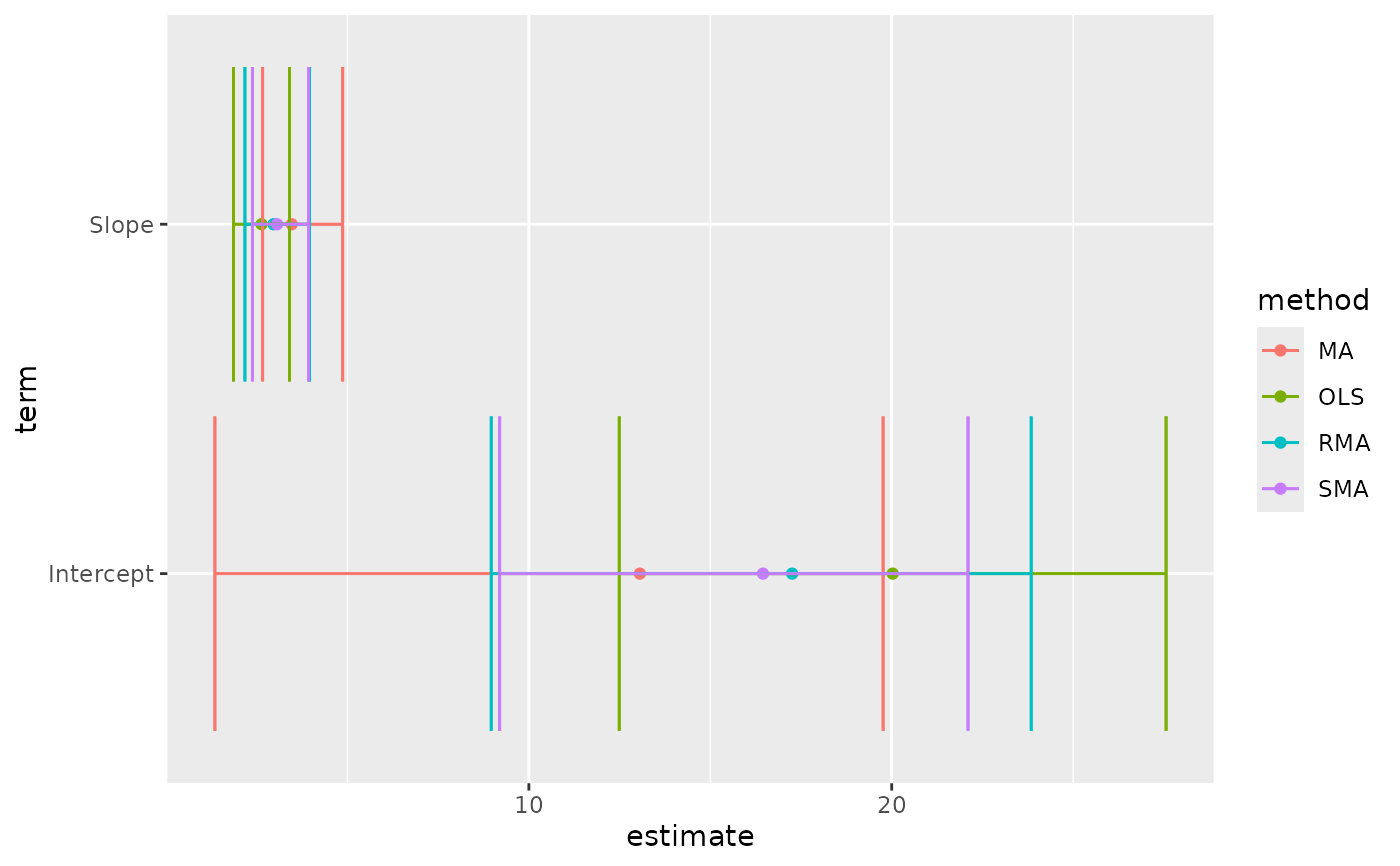Tidy summarizes information about the components of a model. A model component might be a single term in a regression, a single hypothesis, a cluster, or a class. Exactly what tidy considers to be a model component varies across models but is usually self-evident. If a model has several distinct types of components, you will need to specify which components to return.

# S3 method for lmodel2
tidy(x, ...)

## Arguments

x A lmodel2 object returned by lmodel2::lmodel2(). Additional arguments. Not used. Needed to match generic signature only. Cautionary note: Misspelled arguments will be absorbed in ..., where they will be ignored. If the misspelled argument has a default value, the default value will be used. For example, if you pass conf.lvel = 0.9, all computation will proceed using conf.level = 0.95. Additionally, if you pass newdata = my_tibble to an augment() method that does not accept a newdata argument, it will use the default value for the data argument.

## Details

There are always only two terms in an lmodel2: "Intercept" and "Slope". These are computed by four methods: OLS (ordinary least squares), MA (major axis), SMA (standard major axis), and RMA (ranged major axis).

The returned p-value is one-tailed and calculated via a permutation test. A permutational test is used because distributional assumptions may not be valid. More information can be found in vignette("mod2user", package = "lmodel2").

tidy(), lmodel2::lmodel2()

Other lmodel2 tidiers: glance.lmodel2()

## Value

A tibble::tibble() with columns:

conf.high

Upper bound on the confidence interval for the estimate.

conf.low

Lower bound on the confidence interval for the estimate.

estimate

The estimated value of the regression term.

p.value

The two-sided p-value associated with the observed statistic.

term

The name of the regression term.

method

Either OLS/MA/SMA/RMA

## Examples


library(lmodel2)

data(mod2ex2)
Ex2.res <- lmodel2(Prey ~ Predators, data = mod2ex2, "relative", "relative", 99)
Ex2.res
#>
#> Model II regression
#>
#> Call: lmodel2(formula = Prey ~ Predators, data = mod2ex2, range.y =
#> "relative", range.x = "relative", nperm = 99)
#>
#> n = 20   r = 0.8600787   r-square = 0.7397354
#> Parametric P-values:   2-tailed = 1.161748e-06    1-tailed = 5.808741e-07
#> Angle between the two OLS regression lines = 5.106227 degrees
#>
#> Permutation tests of OLS, MA, RMA slopes: 1-tailed, tail corresponding to sign
#> A permutation test of r is equivalent to a permutation test of the OLS slope
#> P-perm for SMA = NA because the SMA slope cannot be tested
#>
#> Regression results
#>   Method Intercept    Slope Angle (degrees) P-perm (1-tailed)
#> 1    OLS  20.02675 2.631527        69.19283              0.01
#> 2     MA  13.05968 3.465907        73.90584              0.01
#> 3    SMA  16.45205 3.059635        71.90073                NA
#> 4    RMA  17.25651 2.963292        71.35239              0.01
#>
#> Confidence intervals
#>   Method 2.5%-Intercept 97.5%-Intercept 2.5%-Slope 97.5%-Slope
#> 1    OLS      12.490993        27.56251   1.858578    3.404476
#> 2     MA       1.347422        19.76310   2.663101    4.868572
#> 3    SMA       9.195287        22.10353   2.382810    3.928708
#> 4    RMA       8.962997        23.84493   2.174260    3.956527
#>
#> Eigenvalues: 269.8212 6.418234
#>
#> H statistic used for computing C.I. of MA: 0.006120651
#>
tidy(Ex2.res)
#> # A tibble: 8 × 6
#>   method term      estimate conf.low conf.high p.value
#>   <chr>  <chr>        <dbl>    <dbl>     <dbl>   <dbl>
#> 1 MA     Intercept    13.1      1.35     19.8     0.01
#> 2 MA     Slope         3.47     2.66      4.87    0.01
#> 3 OLS    Intercept    20.0     12.5      27.6     0.01
#> 4 OLS    Slope         2.63     1.86      3.40    0.01
#> 5 RMA    Intercept    17.3      8.96     23.8     0.01
#> 6 RMA    Slope         2.96     2.17      3.96    0.01
#> 7 SMA    Intercept    16.5      9.20     22.1    NA
#> 8 SMA    Slope         3.06     2.38      3.93   NA   glance(Ex2.res)
#> # A tibble: 1 × 5
#>   r.squared theta    p.value       H  nobs
#>       <dbl> <dbl>      <dbl>   <dbl> <int>
#> 1     0.740  5.11 0.00000116 0.00612    20
# this allows coefficient plots with ggplot2
library(ggplot2)
ggplot(tidy(Ex2.res), aes(estimate, term, color = method)) +
geom_point() +
geom_errorbarh(aes(xmin = conf.low, xmax = conf.high)) +
geom_errorbarh(aes(xmin = conf.low, xmax = conf.high))